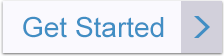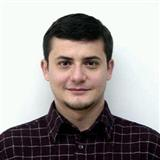Michael BushAway
Tutor in:
Mechanics
Languages:
English

I can provide expert guidance in Algebra, Applied Mathematics, Calculus, Geometry, Linear Algebra, Number Theory, Set Theory, Probability, Statistics and Mechanics.

Degrees

Ph.D. in Mathematical Sciencies
M.S. in Statistics
M.Sc. in Applied Mathematics
Bachelor Degree in Mathematics and Physics

My Tutoring Service

I am a Mathematics Professor with 15 years of teaching experience. I can teach topics related to Algebra, Calculus, Trigonometry, Geometry, Number Theory, Linear Algebra, Statistics, Probability etc. I emphasized more on problem solving skills so that you can understand the concepts related to Mechanics.

Experience & Qualifications

I have over 15 Years of Tutoring experience in various fields of Mathematics like Algebra, Calculus, Geometry, Trigonometry and Statistics. I have developed expertise to solve math problems using Mathematical Software.